# Whx is this an: "improper enumerator cannot be used"?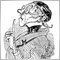Moderator
9919

Hi,

first I tried this - which works fine:

```...
extern ENUM_MA_METHOD      Ma1_Method = 0;
...```

Now I wanted to add (for the tester easy to vary) another not standard moving average, so I wrote:

```enum ma {
SMA =0, // Simple moving average,
EMA =1, // Exponential moving average,
SMMA=2, // Smoothed moving average,
LWMA=3, // Linear weighted moving average.
DEMA=4  // Double Exponential Moving Average.
};
...
extern ma                  Ma1_Method = 0;
...
if ( Ma1_Method < 4 ) {
ma0a = iMA(NULL, 0, Ma1_Period, 0, Ma1_Method, Ma1_Price, shift);
else
ma0d = iCustom(NULL,0,   "DEMA",Ma1_Period,    0,shift);
...```

And this causes the error: "improper enumerator cannot be used" and points to the line of

`ma0a = iMA(NULL, 0, Ma1_Period, 0, Ma1_Method, Ma1_Price, shift);`

Why do I get this error?

What can I do?

Thanks!Moderator
23531

That is strange.

It seems that the enum is being cast as something other than an integer.

If you insert

`   int ma_method=Ma1_Method;`

and then

`    ma0a = iMA(NULL, 0, Ma1_Period, 0, ma_method, Ma1_Price, shift);`

it will compile.21914

gooly: Why do I get this error?
1. ```extern ma                  Ma1_Method = 0;
if ( Ma1_Method < 4 ) {```
If you're going to use enumerations, use them, don't use integers.
2. IMA takes a ENUM_A_METHOD and you are passing a enum ma, so of course you get an error.
3. Take care and properly cast.
 enum ma {    SMA =0, // Simple moving average,    EMA =1, // Exponential moving average,    SMMA=2, // Smoothed moving average,    LWMA=3, // Linear weighted moving average.    DEMA=4  // Double Exponential Moving Average. }; ... extern ma Ma1_Method = 0; ... if ( Ma1_Method < 4 ) {     ma0a = iMA(NULL, 0, Ma1_Period, 0, Ma1_Method, Ma1_Price, shift); else     ma0d = iCustom(NULL,0, "DEMA", Ma1_Period, 0, shift); enum ma {    SMA =MODE_SMA,  // \    EMA =MODE_EMA,  //  \ These must match    SMMA=MODE_SMMA, //  / ENUM_MA for casting    LWMA=MODE_LWMA, // /    DEMA  // < just let it choose next available };       // You shouldn't care what the value is. ... extern ma Ma1_Method = SMA; // < Don't mix ints ... if ( Ma1_Method < DEMA ) {     ma0a = iMA(NULL, 0, Ma1_Period, 0, ENUM_MA_METHOD(Ma1_Method), Ma1_Price, shift);        // cast it from ma to actual ENUM_MA_METHOD else     ma0d = iCustom(NULL,0, "DEMA", Ma1_Period, 0, shift);Moderator
9919

Thank you, WHRoeder!

What you have written should be part of the Help-Documentation.

Such a nice idea, but what a complicated solution.

GoolyModerator
9919

Well I have another solution:

```enum ma {
SMA =MODE_SMA,  // Simple moving average,
EMA =MODE_EMA,  // Exponential moving average,
SMMA=MODE_SMMA, // Smoothed moving average,
LWMA=MODE_LWMA, // Linear weighted moving average.
DEMA            // Double Exponential Moving Average.
};
...
extern ma                  Ma1_Method = SMA;
...
int _Ma1_Meth;
...
int Oninit() {
_Ma1_Meth = Ma1_Method;
...
}
void OnTick(){
ma0a = iMA(NULL, 0, Ma1_Period, 0, _Ma1_Meth, Ma1_Price, x);
...
}```

Again, enum, a nice idea, but what a complicated realisation.Moderator
23531

gooly:

Well I have another solution:

Isn't that the exact same solution that I posted earlier?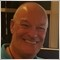307

How to fix this?

Indicator is from Codebase

Thanks

Files: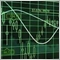2683

Carl Schreiber:

Hi,

first I tried this - which works fine:

Now I wanted to add (for the tester easy to vary) another not standard moving average, so I wrote:

And this causes the error: "improper enumerator cannot be used" and points to the line of

Why do I get this error?

What can I do?

Thanks!

Hi,

just to cast to integer:

` ma0a = iMA(NULL, 0, Ma1_Period, 0, (int)Ma1_Method, Ma1_Price, shift);`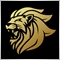Moderator
13063

John Greydanus:

How to fix this?

Indicator is from Codebase

Thanks

No these are improper those are used by other functions.

The identifiers of timeseries are used in the  iHighest() and iLowest() functions. They can be equal to a value the enumeration

ENUM_SERIESMODE

 Identifier Description MODE_OPEN Opening price MODE_LOW Low price MODE_HIGH High price MODE_CLOSE Close price MODE_VOLUME Tick volume MODE_REAL_VOLUME Real volume MODE_SPREAD Spread

In the case of iMA Function:

ENUM_MA_METHOD

 ID Description MODE_SMA Simple averaging MODE_EMA Exponential averaging MODE_SMMA Smoothed averaging MODE_LWMA Linear-weighted averaging

Example:

 double ExtJaws[];double ExtTeeth[];double ExtLips[];//---- handles for moving averagesint    ExtJawsHandle;int    ExtTeethHandle;int    ExtLipsHandle;//--- get MA's handlesExtJawsHandle=iMA(NULL,0,JawsPeriod,0,MODE_SMMA,PRICE_MEDIAN);ExtTeethHandle=iMA(NULL,0,TeethPeriod,0,MODE_SMMA,PRICE_MEDIAN);ExtLipsHandle=iMA(NULL,0,LipsPeriod,0,MODE_SMMA,PRICE_MEDIAN);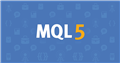Documentation on MQL5: Technical Indicators / iMA
• www.mql5.com
//|                                                     Demo_iMA.mq5 | //|                        Copyright 2011, MetaQuotes Software Corp. | //|                                              https://www.mql5.com | "The method of creation of the handle is set through the 'type' parameter (function type...